# ISEE Middle Level Math : Data Analysis

## Example Questions

### Example Question #1 : How To Find The Answer From A Table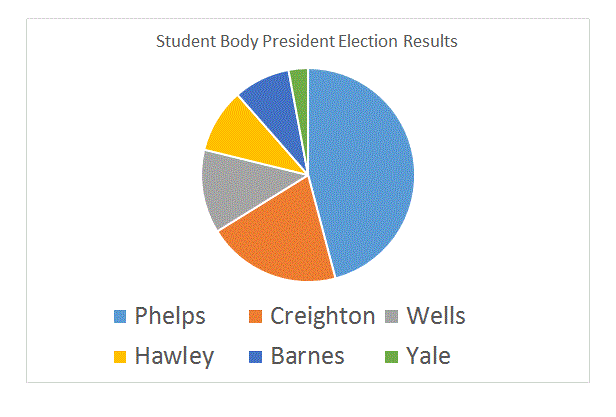The above circle graph shows the results of a school election. According to the rules, the election falls to the student who wins the majority of the votes; if no student wins a majority, the top two vote-getters will face each other in a runoff, with the winner being elected.

Which of the following is the result of this election?

Phelps won the election outright.

None of the other choices is correct.

Phelps and Creighton will face each other in a runoff.

Phelps, Creighton, and Wells will face one another in a runoff.

Phelps and Wells will face each other in a runoff.

Phelps and Creighton will face each other in a runoff.

Explanation:

Since each of the six portions of the graph takes up less than half, no one won a majority. Therefore, there will be a runoff. The two largest portions are light blue (Phelps) and orange (Creighton), so Phelps and Creighton got the most and second-most votes, and they will face each other in a runoff.

### Example Question #1 : TablesThe above circle graph shows the results of a school election. According to the rules, the office of President falls to the student who wins the most votes, and the office of Vice-President falls to the student who finishes second. A runoff only happens in case of a tie.

Which of the following is the result of this election?

Phelps and Creighton will face each other in a runoff to determine which will be President and which will be Vice-President.

Phelps was elected President and Creighton was elected Vice-President.

Phelps and Wells will face each other in a runoff to determine which will be President and which will be Vice-President.

Phelps was elected President and Wells was elected Vice-President.

Phelps was elected President; Creighton and Wells will face each other in a runoff to determine who will be Vice-President.

Phelps was elected President and Creighton was elected Vice-President.

Explanation:

The two largest portions of the pie graph are light blue (Phelps) and orange (Creighton); Phelps got the most votes and Creighton got the second-most. Therefore, Phelps was elected President and Creighton was elected Vice-President - there will be no runoffs.

### Example Question #1 : How To Find The Answer From A TableThe above circle graph shows the results of a school election. According to the rules, the office of President falls to the student who wins the most votes; the office of Vice-President falls to the student who finishes second; the office of Secretary-Treasurer falls to the student who finishes third. A runoff only happens in case of a tie.

Which candidate was elected Secretary-Treasurer?

Hawley was elected Secretary-Treasurer.

Wells and Hawley will face each other in a runoff.

Wells and Creighton will face each other in a runoff.

Wells was elected Secretary-Treasurer.

Creighton was elected Secretary-Treasurer.

Wells was elected Secretary-Treasurer.

Explanation:

The third-largest portion of the circle graph is gray, which, according to the legend, corresponds to Wells's share of the vote. Wells was elected Secretary-Treasurer outright.

### Example Question #7 : Tables

Below is a table that gives the population of Washington City for six census years.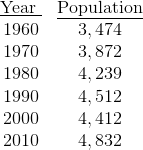Which of the following decades saw the greatest growth in the city's population?

2000 to 2010

1960 to 1970

1970 to 1980

1980 to 1990

2000 to 2010

Explanation:

For each decade, take the difference of the population of the last year and that of the first year. We can omit 1990 to 2000, as the population declined over that decade.

1960-70: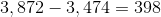1970-80: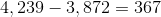1980-90: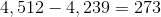2000-10: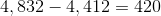The greatest growth occurred between 2000 and 2010.

### Example Question #211 : Data Analysis And Probability

The table below gives the number of students who scored in each of five ranges on a standardized test.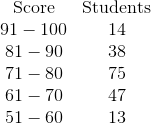No student scored below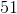.

What percent of the students scoredor less (nearest whole percent)?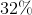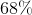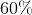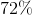Explanation:

Add the students who finished in the bottom two ranges (--and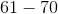):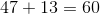Now add all the students from all five ranges: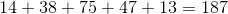To figure out what percent of all of the students finished in the bottom two ranges, calculate: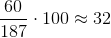The correct choice is.

### Example Question #1 : Tables

Use the following table to answer the question.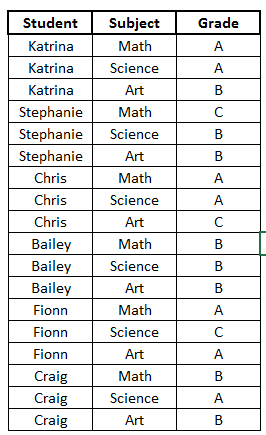How many students received an A in math?Explanation:

When looking at the tablewe can see that is shows several students and within the subjects of Math, Science, and Art what grades those students received in those classes.

So, going over the table briefly, we can see the first student Katrina.  We see for Math she received an A.  For science, Katrina received an A.  And for Art, Katrina got a B.  We can read the table similarly for the remaining students.

Now, the question asks how many students received an A in math.  So we will look through the table and locate Math.  Once we locate Math, we will look at the grade that corresponds with it (In this example, we do not care about who the student is.  We just care about the grade).  So, we will count the number of A's we see matched up to the subject Math.

Once we do that, we see there are 3 students who received A's in Math (Katrina, Chris, and Fionn).

### Example Question #1 : Tables

Use the following table to answer the question.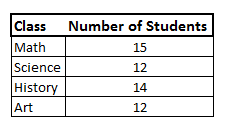What is the total number of students in Math and History?Explanation:

Let's look at the table.we can see the first column is showing the different classes (Math, Science, History, and Art).  The second column shows the number of students in each of those classes.

So, the question is asking how many total students are in Math and History.  We will find the number of students in Math, and find the number of students in History, then add them together.  So,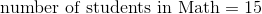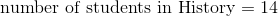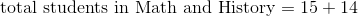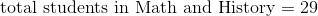### Example Question #111 : Data Analysis

Use the following table to answer the question.What class did Craig have the highest grade in?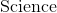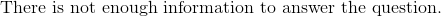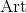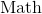Explanation:

Let's look at the table.We can see the first column lists all the students.  The next column shows all of the classes.  And the last column shows the grade they received in those classes.

Now, to find which class Craig received the highest grade, we must first locate Craig.  We can see all of Craig's classes are at the very bottom of the table.

Now, we will look at the grade he got in each class by following along the rows with Craig's name.

We can see Craig's first class is Math.  He received a B.

Craig's second class is Science.  He received an A.

Craig's third class is Art.  He received a B.

Knowing this, we can see the highest grade Craig received was an A.  The class where he received an A was Science.

### Example Question #12 : Tables

Use the following table to answer the question: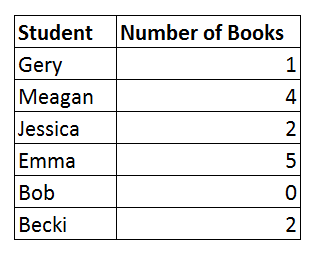How many total books were borrowed by all of the students?Explanation:

To answer the question, let's look at the tableWe can see that the first column shows the students and the second column shows the number of books borrowed by each student.

We want to find the total number of books borrowed by all of the students.  To answer this, we will simply add the number of books borrowed by each student. We get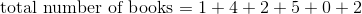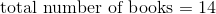Therefore, the total number of books borrowed by all of the students is.

### Example Question #13 : Tables

Use the following table to answer the question: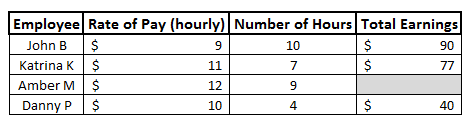Fill in the blank.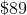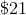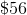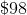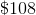Explanation:

To answer the question, let's look at the table.We can see the first column shows the employees.  The next column shows their rate of pay, or how much money they make every hour.  The next column shows how many hours they worked.  And the last column shows their total earning.

So, to find their total earnings, we can see that we multiply the rate of pay by the number of hours.

Therefore, to fill in the blank, we will do the same for that row.  We can see the rate of pay is \$12.  We also see that the number of hours worked is 9.  Now, we will multiply the two numbers together to find the total earnings.  We get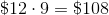Therefore, to fill in the blank, we get.

### All ISEE Middle Level Math Resources